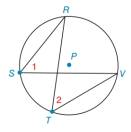Chapter 1.4, Problem 44E### Elementary Geometry for College St...

6th Edition
Daniel C. Alexander + 1 other
ISBN: 9781285195698

#### Solutions

Chapter
Section### Elementary Geometry for College St...

6th Edition
Daniel C. Alexander + 1 other
ISBN: 9781285195698
Textbook Problem
1 views

# Refer to the circle with centre P .a) Use a protractor to find m ∠ 1 .b) Use a protractor to find m ∠ 2 .c) Compare results in parts (a) and (b).To determine

a)

To find:

The measure of 1.

Explanation

Given:

The given figure is a circle with centre P,

Approach:

The given figure is a circle with centre P,

Figure (1)

To determine

b)

To find:

The measure of 2.

To determine

c)

To compare:

The measure of 1 and 2.

### Still sussing out bartleby?

Check out a sample textbook solution.

See a sample solution

#### The Solution to Your Study Problems

Bartleby provides explanations to thousands of textbook problems written by our experts, many with advanced degrees!

Get Started

#### EXPLORING CONCEPTS xx2+1dx

Calculus: Early Transcendental Functions

#### Find the derivative of the function. g(x)=0x4cos(t2)dt

Single Variable Calculus: Early Transcendentals, Volume I

#### A definite integral for the area of the region bounded by y = 2 − x2 and y = x2 is:

Study Guide for Stewart's Single Variable Calculus: Early Transcendentals, 8th